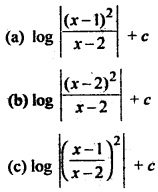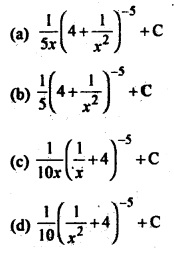# Class 12 Mathematics Chapter 7 Integrals MCQ Questions with Answer

Class 12 Integrals MCQ is one of the best strategies to prepare for the CBSE Class 12 Board exam. If you want to complete a grasp concept or work on one’s score, there is no method except constant practice. Students can improve their speed and accuracy by doing more MCQ on Integrals Class 12, which will help them all through their board test.

## Class 12 Integrals MCQ Questions with Answer

Class 12 Maths MCQ with answers are given here to Chapter 7 Integrals. These MCQs are based on the latest CBSE board syllabus and relate to the latest Class 12 Mathematics syllabus. By Solving these Class 12 MCQs, you will be able to analyze all of the concepts quickly in the chapter and get ready for the Class 12 Annual exam.

Learn Integrals Class 12 MCQ with answers pdf free download according to the latest CBSE and NCERT syllabus. Students should prepare for the examination by solving CBSE Class 12 Mathematics Integrals MCQ with answers given below.

Question 1.
∫1.dx =
(a) x + k
(b) 1 + k
(c) x22 + k
(d) log x + k

A

Question 2. ∫x+sinx1+cosx dx is equal to
(a) log |1 + cos x | + c
(b) log | x + sin x | + c
(c) x – tan + c
(d) x. tan x2 + c

D

Question 3.
∫dx√x =
(a) √x + k
(b) 2√x + k
(c) x + k
(d) 23x3/2 + k

B

Question 4. ∫ba x5 dx =
(a) tan x2 + k
(b) 12 tan x2 + k
(c) 2 tan x2 + k
(d) tan² x2 + k

A

Question 5. If x > a, ∫dxx2−a2 =
(a) 22a log x−ax+a + k
(b) 22a log x+ax−a + k
(c) 1a log(x² – a²) + k
(d) log(x + x2−a2−−−−−−√ + k)

A

Question 6. ∫dx1+cosx =
(a) tan x2 + k
(b) 12 tan x2 + k
(c) 2 tan x2 + k
(d) tan² x2 + k

A

Question 7. ∫10(tan−1x)21+x2 dx =
(a) 1
(b) π264
(c) π2192
(d) None of these

C

Question 8. ∫2−2 |x|dx =
(a) 0
(b) 2
(c) 1
(d) 4

D

Question 9. ∫cos2xdx(sinx+cosx)2 =
(a) –1sinx+cosx + c
(b) log | sin x + cos x | + c
(c) log | sin x – cos x | + c
(d) 1(sinx+cosx)2

B

Question 10. ∫(1+logx)21+x2 dx =
(a) 13(1+log)³ + c
(b) 12(1+log)² + c
(c) log (log 1 + x) + 2
(d) None of these

A

Question 11. ∫sin2x−cos2xsin2xcos2x dx is equal to
(a) tan x + cos x + c
(b) tan x + cosec x + c
(c) tan x + cot x + c
(d) tan x+ sec x + c

C

Question 12. ∫x4+1×2+1 dx is equal to
(a) x33 + x + tan-1 x + c
(b) x33 – x + tan x + c
(c) x33 + x + 2tan-1 x + c
(d) x33 – x + 2tan-1 x + c

D

Question 13. ∫(√x + 1√x) dx =
(a) 1x x13 + 2x12 + c
(b) 23 x23 + 12x2 + c
(c) 23 x32 + 2x12 + c
(d) 32 x32 + 12x12 + c

C

Question 14. What is the value of ∫π/20 tanx√tanx√+cotx√ dx?
(a) π2
(b) π4
(c) π8
(d) None of these

B

Question 15. ddx∫f(x)dx is equal to
(a) f'(x)
(b) f(x)
(c) f'(x’)
(d) f(x) + c

B

Question 16. ∫xdx(x−1)(x−2) equals(d) log |(x – 1)(x – 2) + c

B

Question 17. What is the value of ∫1−1 sin³ x cos² xdx?
(a) 0
(b) 1
(c) 12
(d) 2

A

Question 18. What is the value of ∫π/20 sinx–cosx1+sinxcosx dx?
(a) 1
(b) π2
(c) 0
(d) –π2

C

Question 19. What is the value of ∫π/3π/6 dxsin2x?
(a) 12 log(-l)
(b) log(- 1)
(c) log 3
(d) log √3

C

Question 20. ∫π2/40siny√y√
(a) 1
(b) 2
(c) π4
(d) π28

B

Question 21. What is the value of ∫e11+logxx dx?
(a) 32
(b) 12
(c) e
(d) 1e

A

Question 22. ∫π/2−π/2 sin9 xdx =
(a) -1
(b) 0
(c) 1
(d) None of these

B

Question 23. What is the value of ∫10 ddx{sin-1(2×1+x2)}dx?
(a) 0
(b) π
(c) -π
(d) π2

D

Question 24. ∫∞011+ex dx =
(a) log 2
(b) -log 2
(c) log 2 – 1
(d) log 4 – 1

A

Question 25. ∫10 x(1 – x)99 is equal to
(a) 110010
(b) 110100
(c) 11010
(d) 1110100

B

Question 26. ∫log10 xdx =
(a) loge 10.x loge (xe) + c
(b) log10 e.x loge (xe) + c
(c) (x – 1) loge x + c
(d) 1x + c

B

Question 27. ∫10 x1+x dx =
(a) 1 – log 2
(b) 2
(c) 1 + log 2
(d) log 2

A

Question 28. ∫sinx+cosx1+2sinx√ dx =
(a) log(sin x – cos x)
(b) x
(c) log x
(d) log sin (cos x)

B

Question 29. Value of ∫dx2x–x2√
(a) sin-1 (x – 1) + c
(b) sin-1 (1 + x) + c
(c) sin-1 (1 + x²) + c
(d) –2x−x2−−−−−−√ + c

A

Whoever needs to take the CBSE Class 12 Board Exam should look at this MCQ. To the Students who will show up in CBSE Class 12 Mathematics Board Exams, It is suggested to practice more and more questions. Aside from the sample paper you more likely had solved. These Integrals Class 12 MCQ are ready by the subject specialists themselves.

Question 30. ∫(cos2θ–1cos2θ+1) dθ =
(a) tan θ – θ + c
(b) θ + tan θ + c
(c) θ – tan θ + c
(d) -θ – cot θ + c

C

Question 31. ∫2dx1−4×2√ =
(a) tan-1 (2x) + c
(b) cot-1 (2x) + c
(c) cos-1 (2x) + c
(d) sin-1 (2x) + c

D

Question 32. ∫dxsin(x−a)sin(x−b) is equal to
(a) sin(b – a) log |sin(x−b)sin(x−a)| + C
(b) cosec (b – a) log |sin(x−b)sin(x−b)| + C
(c) cosec (b – a) log |sin(x−b)sin(x−a)| + C
(d) sin (b – a) log |sin(x−a)sin(x−b)| + C

C

Question 33. ∫tan-1 √xdx is equal to
(a) (x + 1)tan-1 √x – √x + C
(b) x tan-1 √x – √x + C
(c) √x – x tan-1 √x + C
(d) √x – (x + 1)tan-1 √x + C

A

Question 34. ∫x² sin x³ dx =
(a) 13 cos x³ + c
(b) –13 cos x + c
(c) −13 cos x³ + c
(d) 12 sin² x³ + c

C

Question 35. ∫cos2x−cos2θcosx–cosθdx is equal to
(a) 2 (sin x + x cos θ) + C
(b) 2 (sin x – x cos θ) + C
(c) 2 (sin x + 2x cos θ) + C
(d) 2 (sin x – 2x cos θ) + C

A

Question 36. If ∫dx(x+2)(x2+1) = a log |1 + x²| + b tan-1 x + 15 log |x + 2| + C, then
(a) a = −110, b = −25
(b) a = 110, b = −25
(c) a = −110, b = 25
(d) a = 110, b = 25

C

Question 37. ∫ex(1−x1+x2)² dx is equal to
(a) ex1+x2 + C
(b) –−ex1+x2 + C
(c) ex(1+x2)2 + C
(d) −ex(1+x2)2 + C

A

Question 38. ∫x9(4×2+1)6 dx is equal toD

Question 39. ∫π/4−π/4 dx1+cos2x dx is equal to
(a) 1
(b) 2
(c) 3
(d) 4

A

Question 40. ∫ x3x+1 is equal to
(a) x + x22 + x33 – log |1 – x| + C
(b) x + x22 – x33 – log |1 – x| + C
(c) x + x22 – x33 – log |1 + x| + C
(d) x + x22 + x33 – log |1 + x| + C

D

Question 41. If ∫x3dx1+x2√ = a(1 + x²)3/2 + b1+x2−−−−−√ + C, then
(a) a = 13, b = 1
(b) a = −13, b = 1
(c) a = −13, b = -1
(d) a = 13, b = -1

D

Question 42. Evaluate: ∫ sec²(7 – 4x)dx
(a) –14 tan(7 – 4x) + C
(b) 14 tan(7 – 4x) + C
(c) 14 tan(7 + 4x) + C
(d) –14 tan(7x – 4) + C

A

Question 43. ∫π/20 1+sin2x−−−−−−−−√ dx is equal to
(a) 2√2
(b) 2(√2 + 1)
(c) 0
(d) 2(√2 – 1)

C

Question 44. Evaluate: ∫(2 tan x – 3 cot x)² dx
(a) -4tan x – cot x – 25x + C
(b) 4 tan x – 9 cot x – 25x + C
(c) – 4 tan x + 9 cot x + 25x + C
(d) 4 tan x + 9 cot x + 25x + C

B

Question 45. ∫ a(1+x2)tan−1x dx =
(a) a log |tan-1 x| + C
(b) 12(tan-1 x)² + C
(c) a log (1 + x2) + C
(d) None of these

A

Question 46. ∫ 10×9+10xloge1010x+x10 dx is equal to
(a) 10x – x10 + C
(b) 10x + x10 + C
(c) (10x – x10)-1 + C
(d) loge(10x + x10) + C

D

Question 47. Evaluate: ∫ sec4/3 x cosec8/3 xdx
(a) 35 tan-5/3 x – 3 tan1/3 x + C
(b) –35 tan-5/3 x + 3 tan1/3 + C
(c) –35 tan-05/3 x – 3 tan1/3 + C
(d) None of these

B

Question 48. ∫ cotxsinx√3 dx =
(a) −3sinx√3 + C
(b) −2sin3x + C
(c) 3sin1/3x + C
(d) None of these

A

Question 49. Evaluate: ∫ 19+8x−x2√ dx
(a) -sin-1 (x−45) + C
(b) sin-1 (x+45) + C
(c) sin-1 (x−45) + C
(d) None of these

C

Question 50. ∫ dx1−cosx−sinx is equal to
(a) log |1 + cotx2| + C
(b) log |1 – tanx2| + C
(c) log |1 – cotx2| + C
(d) log |1 + tanx2| + C

C

Question 51. Evaluate: ∫ 11+3sin2x+8cos2x dx
(a) 16 tan-1 (2 tan x) + C
(b) tan-1 (2 tan x) + C
(c) 16 tan-1(2tanx3) + C
(d) None of these

C

Question 52. If ∫ 3x+4×3−2x−4 dx = log |x – 2| + k log f(x) + c, then
(a) f(x) = |x² + 2x + 2|
(b) f(x) = x² + 2x + 2
(c) k = –12
(d) All of these

D

Question 53. Evaluate: ∫ 11−e2x√ dx
(a) log |e-x + e−2x–1−−−−−−√| + C
(b) -log |e-x + e−2x–1−−−−−−√| + C
(c) -log |e-x – e−2x–1−−−−−−√| + C
(d) None of these

B

Question 54. ∫ cos(loge.x)dx is equal to
(a) 12 x[cos (logex) + sin(logex)]
(b) x[cos (logex) + sin(logex)]
(c) 12 x[cos (logex) – sin(logex)]
(d) x[cos (logex) – sin(logex)]

B

Question 55. ∫ |x| dx is equal to
(a) 12 x² + C
(b) –x22 + C
(c) x|x| + C
(d) 12 x|x| + C

D

Question 56. Evaluate: ∫ 1−cosxcosx(1+cosx) dx
(a) log|sec x + tan x| – 2 tan(x/2) + C
(b) log|sec x – tan x| – 2 tan(x/2) + C
(c) log|sec x + tan x| + 2 tan(x/2) + C
(d) None of these

A

Question 57. ∫ cos-1(1x)dx equals
(a) x sec-1 x + log |x + x2−1−−−−−√| + C
(b) x sec-1 x – log |x + x2−1−−−−−√| + C
(c) -x sec-1 x – log |x + x2−1−−−−−√| + C
(d) None of these

B

Question 58. ∫ sin-1 xdx is equal to
(a) cos-1 x + C
(b) x sin-1x + 1−x2−−−−−√ + C
(c) 11−x2√ + C
(d) x sin-1x – 1−x2−−−−−√ + C

B

You can easily get good marks If you study with the help of Class 12 Integrals MCQ. We trust that information provided is useful for you. NCERT MCQ Questions for Class 12 Integrals PDF Free Download would without a doubt create positive results.

We hope the information shared above in regards to MCQ on Integrals Class 12 with Answers has been helpful to you. if you have any questions regarding CBSE Class 12 Integrals MCQ Pdf, write a comment below and we will get back to you as soon as possible.